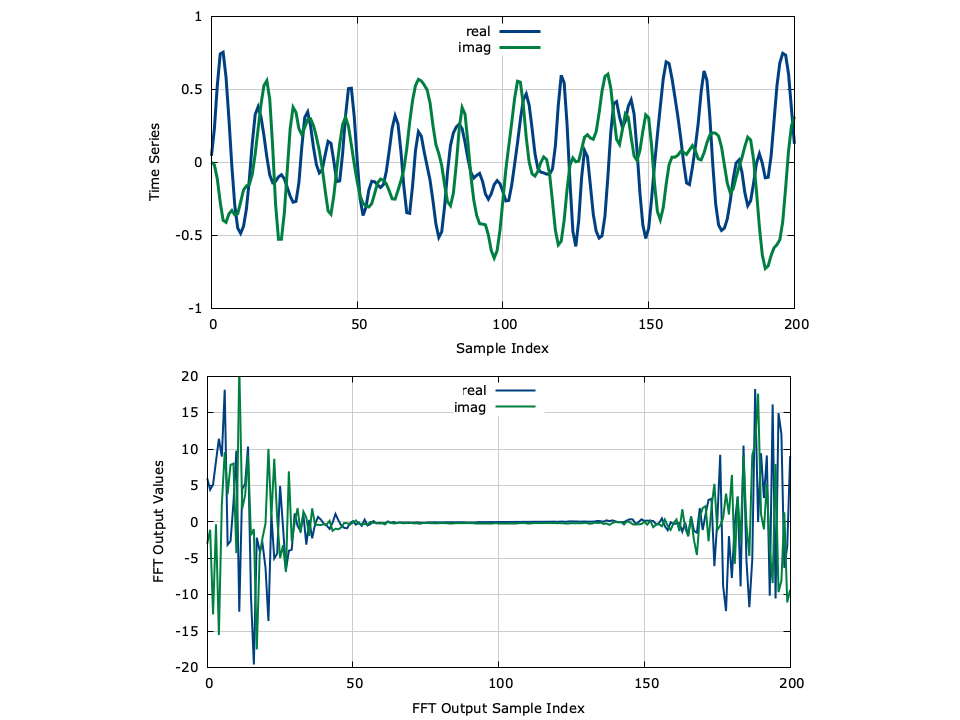# Fast Fourier Transform (fft)

The fft module in liquid implements fast discrete Fourier transforms including forward and reverse DFTs as well as real even/odd transforms.

## Complex Transforms

Given a vector of complex time-domain samples $$\vec{x} = \left[x(0),x(1),\ldots,x(N-1)\right]^T$$ the $$N$$ -point forward discrete Fourier transform is computed as:

$$X(k) = \sum_{i=0}^{N-1}{x(i) e^{-j 2 \pi k i/N}}$$

Similarly, the inverse (reverse) discrete Fourier transform is:

$$x(n) = \sum_{i=0}^{N-1}{X(i) e^{ j 2 \pi n i/N}}$$

Internally, liquid uses several algorithms for computing FFTs including the standard decimation-in-time (DIT) for power-of-two transforms {cite:Ziemer:1998(Section 10-4)}, the Cooley-Tukey mixed-radix method for composite transforms {cite:CooleyTukey:1965}, Rader's algorithm for prime-length transforms {cite:Rader:1968}, and the DFT given by [eqn-fft-dft] for very small values of $$N$$ . The DFT requires $$\ord\bigl(N^2\bigr)$$ operations and can be slow for even moderate sizes of $$N$$ which is why it is typically reserved for small transforms. liquid 's strategy for computing FFTs is to recursively break the transform into manageable pieces and perform the best method for each step. For example, a transform of length $$N=128=2^7$$ can be easily computed using the standard DIT FFT algorithm which is computationally fast. The Cooley-Tukey algorithm permits any factorable transform of size $$N=PQ$$ to be computed with $$P$$ transforms of size $$Q$$ and $$Q$$ transforms of size $$P$$ . For example, a transform of length $$N=126$$ can be computed using the Cooley-Tukey algorithm with radices $$P=9$$ and $$Q=14$$ . Furthermore, each of these transforms can be further split using the Cooley-Tukey algorithm (e.g. $$9=3\cdot3$$ and $$14=2\cdot7$$ ). The smallest resulting transforms can finally be computed using the DFT algorithm without much penalty. For large transforms of prime length, liquid uses Rader's algorithm {cite:Rader:1968} which permits any transform of prime length $$N$$ to be computed using an FFT and an IFFT each of length $$N-1$$ . For example, Rader's algorithm can compute a 127-point transform using the 126-point Cooley-Tukey transform (and its inverse) described above.


.. footnote

Rader actually gives an alternate algorithm by which any
transform of prime length $N$ can be computed with an FFT and
an IFFT of any length greater than $2N-4$.
For example, the 127-point FFT could also be computed using
computationally efficient 256-point DIT transforms.
liquid includes both algorithms and chooses the most
appropriate one for the task.


Through recursion, a tranform of any size can be decomposed into either computationally efficient DIT FFTs, or combinations of small DFTs. Consequently, liquid can compute any transform in $$\ord\bigl(n\log(n)\bigr)$$ operations.

Even still, liquid will use the fftw3 library library {cite:fftw:web} for internal methods if it is available. The presence of fftw3.h and libfftw3 are detected by the configure script at build time. If found, liquid will link against fftw for better performance (it is, however, the fastest FFT in the west, you know). If fftw is unavailable, however, liquid will use its own, slower FFT methods for internal processing. This eliminates libfftw as an external dependency, but takes advantage of it when available.

An example of the interface for computing complex discrete Fourier transforms is listed below. Notice the stark similarity to libfftw3 's interface.


#include <liquid/liquid.h>

int main() {
// options
unsigned int n=16;  // input data size
int flags=0;        // FFT flags (typically ignored)

// allocated memory arrays
float complex * x = (float complex*) malloc(n * sizeof(float complex));
float complex * y = (float complex*) malloc(n * sizeof(float complex));

// create FFT plan
fftplan q = fft_create_plan(n, x, y, LIQUID_FFT_FORWARD, flags);

// ... initialize input ...

// execute FFT (repeat as necessary)
fft_execute(q);

// destroy FFT plan and free memory arrays
fft_destroy_plan(q);
free(x);
free(y);
}


## ExampleFigure [fig-fft-example]. Example FFT

## Real even/odd DFTs

liquid also implement real even/odd discrete Fourier transforms; however these are not guaranteed to be efficient. A list of the transforms and their descriptions is given below.

### FFT_REDFT00 (DCT-I)

$$X(k) = \frac{1}{2}\Bigl( x(0) + (-1)^k x(N-1) \Bigr) + \sum_{n=1}^{N-2}{x(n) \cos\left(\frac{\pi}{N-1}nk\right) }$$

### FFT_REDFT10 (DCT-II)

$$X(k) = \sum_{n=0}^{N-1}{ x(n) \cos\left[ \frac{\pi}{N}\left(n + 0.5\right)k \right] }$$

### FFT_REDFT01 (DCT-III)

$$X(k) = \frac{x(0)}{2} + \sum_{n=1}^{N-1}{ x(n) \cos\left[ \frac{\pi}{N}n\left(k + 0.5\right) \right] }$$

### FFT_REDFT11 (DCT-IV)

$$X(k) = \sum_{n=0}^{N-1}{ x(n) \cos\left[ \frac{\pi}{N} \left(n+0.5\right) \left(k+0.5\right) \right] }$$

### FFT_RODFT00 (DST-I)

$$X(k) = \sum_{n=0}^{N-1}{ x(n) \sin\left[ \frac{\pi}{N+1}(n+1)(k+1) \right] }$$

### FFT_RODFT10 (DST-II)

$$X(k) = \sum_{n=0}^{N-1}{ x(n) \sin\left[ \frac{\pi}{N}(n+0.5)(k+1) \right] }$$

### FFT_RODFT01 (DST-III)

$$X(k) = \frac{(-1)^k}{2}x(N-1) + \sum_{n=0}^{N-2}{ x(n) \sin\left[ \frac{\pi}{N}(n+1)(k+0.5) \right] }$$

### FFT_RODFT11 (DST-IV)

$$X(k) = \sum_{n=0}^{N-1}{ x(n) \sin\left[ \frac{\pi}{N}(n+0.5)(k+0.5) \right] }$$

An example of the interface for computing a discrete cosine transform of type-III ( FFT_REDFT01 ) is listed below.


#include <liquid/liquid.h>

int main() {
// options
unsigned int n=16;              // input data size
int type = LIQUID_FFT_REDFT01;  // DCT-III
int flags=0;                    // FFT flags (typically ignored)

// allocated memory arrays
float * x = (float*) malloc(n * sizeof(float));
float * y = (float*) malloc(n * sizeof(float));

// create FFT plan
fftplan q = fft_create_plan_r2r_1d(n, x, y, type, flags);

// ... initialize input ...

// execute FFT (repeat as necessary)
fft_execute(q);

// destroy FFT plan and free memory arrays
fft_destroy_plan(q);
free(x);
free(y);
}